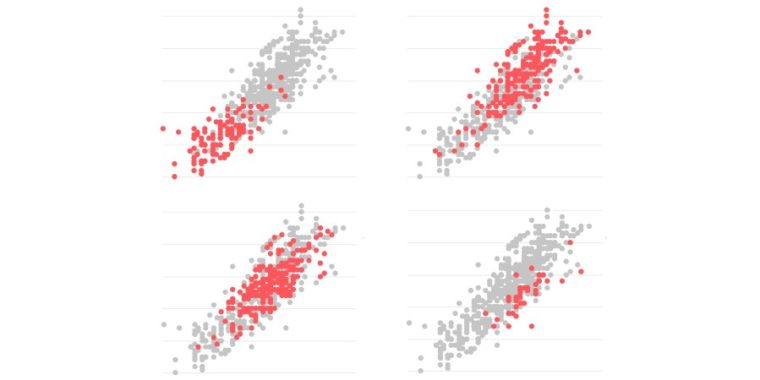# Interactive Small Multiple with RFinding a balance between broad-strokes vs. a detailed data visualization approach can be challenging, especially if either approach obscures as much as it illuminates the data story. However, there is an alternate path: In this article, we will look at visualizing data for comparison purposes, and how highlighting a select group of data within a larger data-set allows for both a high-level and a granular view.

Remark

In the previous article, we explored the power of combining a box plot with a jitter. This combination allows us to compare one continuous variable and visualize its size for each discipline. Nevertheless, if you want to explore the comparison of data sets using a relationship between two continuous variables, your best option is to use small multiple to highlight one data set at the time. For example, the charts below visualize the relationship between height and weight (men) for the following disciplines: Gymnastics, Modern Pentathlon, Canoe, and Hockey (see below).

Here is the code for one chart:

```library("highcharter")
library("dplyr")
library("plyr")

cols <- c("#c5c5c5", "#ff5858")

df <-
"https://raw.githubusercontent.com/mekhatria/demo_highcharts/master/Olympics2012CapitalLetter.csv"
)
#Gather the disciplines under the name Additional to compare as one series with the targeted discipline
df\$sport <-
revalue(
df\$sport,
c(
)
)
#Remove the unnecessary data such as nationality, date of birth, name, and age
df = subset(df, select = -c(nationality, date_of_birth, name, age))
#Filter only the targeted and additional disciplines using the discipline names and sex (male in this case)
my_data <- df %>% filter((sport == "Gymnastics" &
sex == "male") |  (sport == "Additional" & sex == "male"))
#Remove the redundant data
my_data = subset(my_data, select = -c(sex))
#Create the chart
hchart(my_data, "scatter", hcaes(x = height, y = weight, group = sport)) %>%
hc_title(text = "Gymnastics") %>%
hc_xAxis(title = list(text = ""),
labels = list(format = "{value} m")) %>%
hc_yAxis(title = list(text = ""),
labels = list(format = "{value} kg")) %>%
hc_tooltip(useHTML = TRUE,